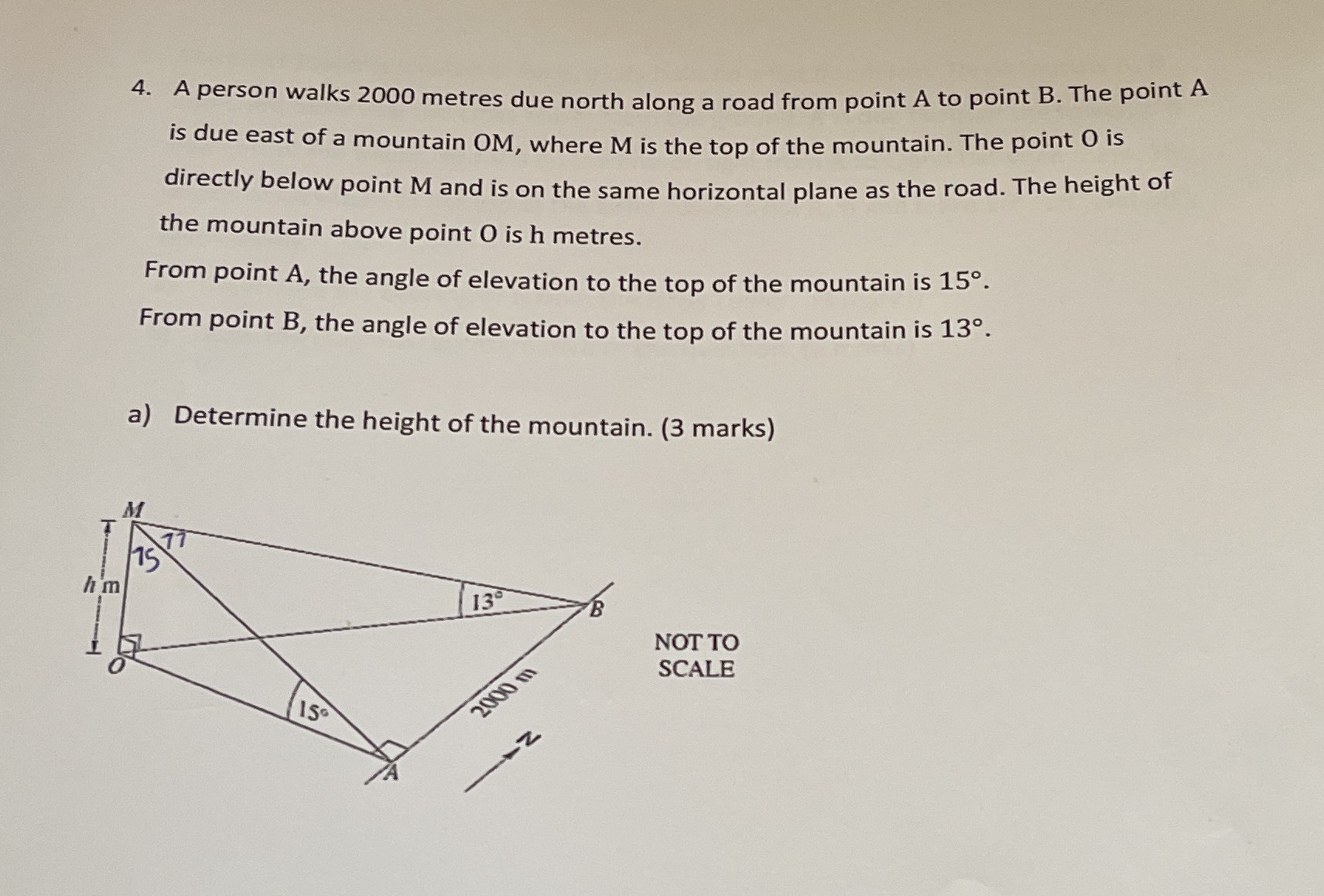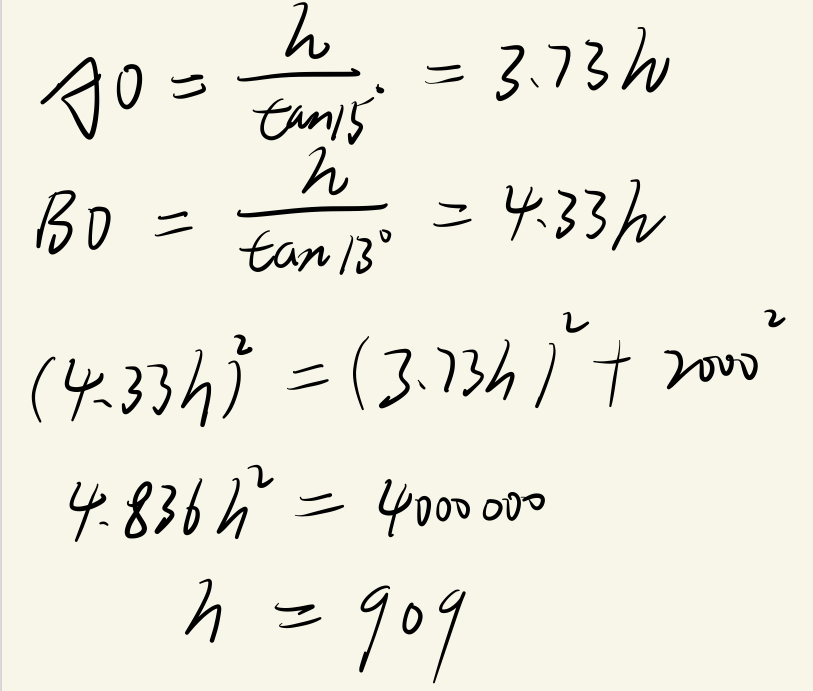### ¿Todavía tienes preguntas de matemáticas?

Pregunte a nuestros tutores expertos
Algebra
Pregunta4. A person walks $$2000$$ metres due north along a road from point $$A$$ to point $$B$$ . The point $$A$$ is due east of a mountain oM, where $$M$$ is the top of the mountain. The point $$0$$ is directly below point $$M$$ and is on the same horizontal plane as the road. The height of the mountain above point $$O$$ is h metres. From point $$A$$ , the angle of elevation to the top of the mountain is $$15 ^ { \circ }$$ . From point $$B$$ , the angle of elevation to the top of the mountain is $$13 ^ { \circ }$$ .

a) Determine the height of the mountain. (3 marks)